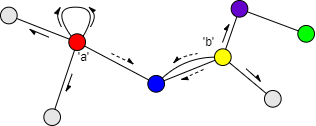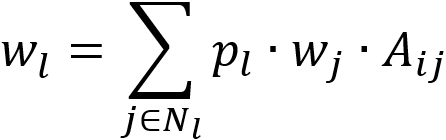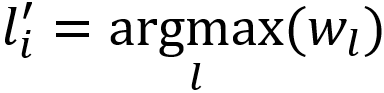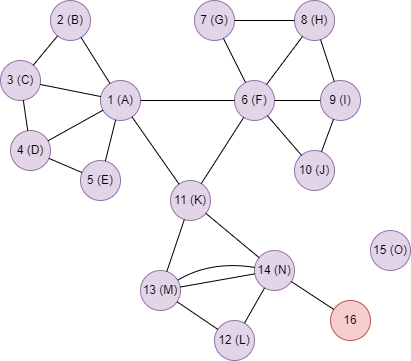# Change Nickname

Current Nickname:

Certifications

Certificate Issued at Valid until Serial No. File
Serial No. Valid until

Not having one? Apply now! >>>

Invoice

ProductName CreateTime ID Price File
ProductName CreateTime ID Price File

# Label Propagation

## Overview

Label Propagation Algorithm (LPA) is a community detection algorithm based on label propagation; it is an iterative process of nodes aggregation with the goal of label distribution stabilization. Although the results of the algorithm have a certain degree of randomness, it is very suitable for large complex networks which owes to its low time complexity and no need to specify the number of communities in advance. It is often included in the benchmark testing report in both academia and industry.

Related material of the algorithm:

## Basic Concept

### Label

Label is the value of a specified property of node. Label can be propagated to the 1-step neighbors of the node, nodes with label can adopt label from its 1-step neighbors. When detecting the communities, label represents the community of the node, the propagation of label is the expansion of the community.Consider the propagation of label `a` and `b` of the red and yellow nodes in the graph above:

• Blue node is the common neighbor of the two nodes, it will decide which label to adopt based on the weight of label `a` and `b`, or to adopt both labels if it is allowed.
• Red node has self-loop edge, so label `a` also propagates to itself.
• Label `b` cannot propagate to the green node during one round of propagation; if the purple node (green node's only neighbor) adopts label `b`, the green node will adopt label `b` during the next round of propagation.

Ultipa's LPA supports full label propagation, that is, all initial labels are valid and able to propagate. In case there is a need to specify part of the labels as valid, please contact Ultipa team for algorithm customization.

### Label Probability

If a node can only adopt only one label from its neighbors, it is called single-label propagation, while if multiple labels are allowed to adopt, it is called multi-label propagation.

In multi-label propagation, after a node adopts multiple labels, each label will be given a probability which is proportional to the label weight, and the sum of all label probabilities of a node equals to 1. For single-label propagation, the case is much easier as the node's only label takes a probability of 1.

For example, if a node only adopts one label, the label probability is `1`; if it adopts labels `a` and `b` with weights `1.5` and `1` respectively, the probabilities of `a` and `b` are `1.5/(1+1.5) = 0.6` and `1/(1+1.5) = 0.4` respectively.

### Label Weight

Node `j` has label `l`, when it propagates to its neighbor node `i`, the weight of label `l` equals to the product of the probability of `l`, the weight of `j`, and the total weight of all edges between `i` and `j`; when `i` has multiple neighbor nodes with label `l`, the weight of each `l` needs to be summed:where `j` is any neighbor node of `i` that has label `l` , `p(l)` is the probability of `l`, `w(j)` is the weight of `j`, `A(ij)` is the total weight of all edges between `i` and `j`.

It is needed to specify a numeric property of node as node weight, and a numeric property of edge as edge weight.

The principle of label adoption is to keep the top one or several labels with the largest weights among the neighbor labels, and to calculate the label probabilities. When some labels have the same probabilities which affects the adoption decision, the system would select randomly from these parallel labels.

For any node `i` in the graph, `l` is the neighbor label of `i`, `w(l)` is the weight of `l`, the new label `l` of `i` can be denoted as:As mentioned previously, under the circumstances of multi-label propagation, the algorithm normalizes the label weight, that is, converts label weight into a weight probability.

When the algorithm begins, initial labels are assigned to nodes based on the algorithm parameters; during each iteration, it calculates for each node whether there is any label different from its current labels can be adopted from its neighbors, and after all nodes are calculated, updates the nodes which can adopt new labels. Iterating and looping by this rule until no node could adopt new labels, or the number of iterations reaches the limit.

Ultipa's LPA follows the synchronous update principle when updating node labels, the algorithm has a corresponding interrupt mechanism against the label oscillations (mostly found in bipartite graph) that might occur.

## Special Case

### Lonely Node, Disconnected Graph

For disconnected graph, there is no edge between its lonely nodes and connected components to propagate labels, connected components do not contain the same initial labels will not be divided into the same community.

### Self-loop Edge

LPA treats the self-loop edge in the same way with the Degree algorithm `algo(degree)`, which counts each self-loop edge twice.

### Directed Edge

For directed edges, LPA ignores the direction of edges but calculates them as undirected edges.

## Results and Statistics

Run LPA in the graph below, all node and edge weights are 1, the letter wrote in the node is the initial label of node, node 16 has no label, the algorithm iterates 5 rounds:Algorithm results 1: Keep maximum one label for each node, return `_uuid`, `label_1` and `probability_1`

_uuid label_1 probability_1
1 C 1.000000
2 C 1.000000
3 C 1.000000
4 C 1.000000
5 C 1.000000
6 H 1.000000
7 H 1.000000
8 H 1.000000
9 H 1.000000
10 H 1.000000
11 N 1.000000
12 N 1.000000
13 N 1.000000
14 N 1.000000
15 O 1.000000
16

Algorithm statistics 1: Number of labels `label_count`

label_count
4

Algorithm results 2: Keep maximum two labels for each node, return `_uuid`, `label_1`, `probability_1`, `label_2` and `probability_2`

_uuid label_1 probability_1 label_2 probability_2
1 C 0.705578 A 0.294422
2 C 0.658854 A 0.341145
3 A 0.508263 C 0.491737
4 C 0.688864 E 0.311136
5 A 0.572178 C 0.427822
6 H 0.723903 A 0.276097
7 H 0.797693 I 0.202307
8 H 0.645654 I 0.354346
9 H 0.779780 A 0.220220
10 H 0.617815 I 0.382185
11 N 0.611782 M 0.388218
12 N 0.611782 M 0.388218
13 N 0.766861 M 0.233139
14 N 0.663694 M 0.336306
15 O 1.000000
16

Algorithm statistics 2: Number of labels `label_count`

label_count
8

## Command and Configuration

• Command: `algo(lpa)`
• Configurations for the parameter `params()`:
Name Type
Default
Specification Description
node_label_property `@<schema>.<property>` / Numeric or string class node property, LTE needed Name of the node schema and property as label; node without this property will not participate in the calculation; random number is used as label if not set
node_weight_property `@<schema>.<property>` / Numeric node property, LTE needed Name of the node schema and property as node weight; node without this property will not participate in the calculation; weight is 1 if not set
edge_weight_property `@<schema>.<property>` / Numeric edge property, LTE needed Name of the edge schema and property as edge weight; edge without this property will not participate in the calculation; weight is 1 if not set
k int 1 >=1 The maximum number of labels each node can keep; labels are ordered by probability
loop_num int 5 >=1 Number of iterations

Example: Run LPA, label locates on node property @default.name, iterate 5 rounds and keep no more than two labels for each node

``````algo(lpa).params({
node_label_property: @default.name,
k: 2,
loop_num: 5
}) as a,b return a,b
``````

## Algorithm Execution

#### 1. File Writeback

Configuration
Data in Each Row
filename `_id`,`label_1`,`probability_1`,...`label_k`,`probability_k`

Example: Run LPA, label locates on node property @default.name, iterate 5 rounds and keep no more than two labels for each node, write the algorithm results back to file named lpa

``````algo(lpa).params({
node_label_property: @default.name,
k: 2,
loop_num: 5
}).write({
file:{
filename: "lpa"
}
})
``````

#### 2. Property Writeback

Configuration
Writeback Content
Type
Data Type
property `label_1`, `probability_1`, ... `label_k`, `probability_k` Node property Data type of label is `string`, data type of label probability is `float`

Example: Run LPA, label locates on node property @default.name, iterate 5 rounds and keep no more than two labels for each node, write the algorithm results back to node properties named newName_1, probability_1, newName_2 and probability_2

``````algo(lpa).params({
node_label_property: @default.name,
k: 2,
loop_num: 5
}).write({
db:{
property: "newName"
}
})
``````

#### 3. Statistics Writeback

Name Data Type Description
`label_count` int Number of labels

The number of labels means the number of labels left after the algorithm is finished; when k = 1, since each node only keeps one label, the number of labels is the number of communities.

Example: Run LPA, label locates on node property @default.name, iterate 5 rounds and keep no more than two labels for each node, write the algorithm statistics back to task information

``````algo(lpa).params({
node_label_property: @default.name,
k: 2,
loop_num: 5
}).write()
``````

### Direct Return

Alias Ordinal
Type
Description Column Name
0 []perNode Node and its label, label probability `_uuid`, `label_1`, `probability_1`, ... `label_k`, `probability_k`
1 KV Number of labels `label_count`

Example: Run LPA, label locates on node property @default.name, iterate 5 rounds and keep no more than one label for each node, define algorithm results and statistics as aliases named results and count, and return the results and statistics

``````algo(lpa).params({
node_label_property: @default.name,
k: 1
}) as results, count
return results, count
``````

### Streaming Return

Alias Ordinal
Type
Description Column Name
0 []perNode Node and its label, label probability `_uuid`, `label_1`, `probability_1`, ... `label_k`, `probability_k`

Example: Run LPA, label locates on node property @default.name, iterate 5 rounds and keep no more than one label for each node, and return the results ordered by the ascending label name and node UUID

``````algo(lpa).params({
node_label_property: @default.name,
k: 1,
loop_num: 5
}).stream() as lpa
return lpa order by lpa.label_1, lpa._uuid
``````

### Real-time Statistics

Alias Ordinal
Type
Description Column Name
0 KV Number of labels `label_count`

Example: Run LPA, label locates on node property @default.name, iterate 5 rounds and keep no more than one label for each node, and return the number of communities

``````algo(lpa).params({
node_label_property: @default.name,
k: 1,
loop_num: 5
}).stats() as count
return count
``````

## Consistency of Results

Affected by factors such as the order of nodes, the random selection of labels with the same weights, the parallel calculation and so on, the community division results of LPA may be different.